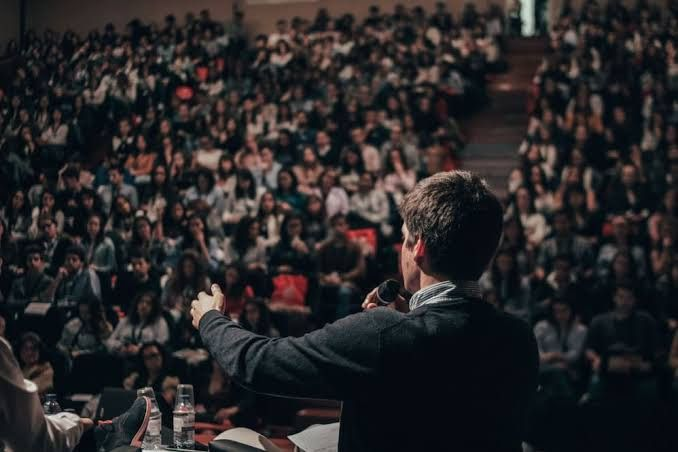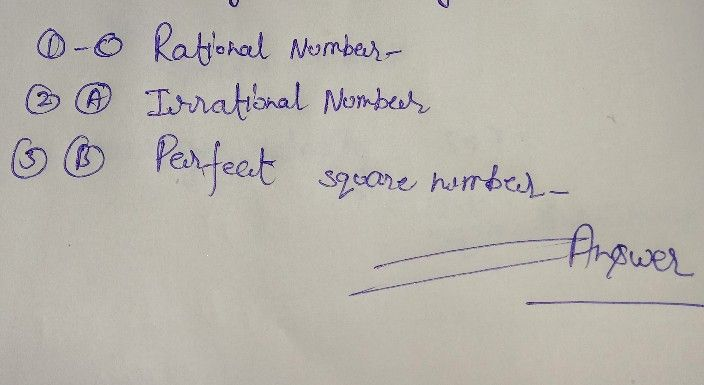Symbol
ProblemMuhge $.sx2$ Wite the letter of your answer on a separate answer sheet. 1 A is a mumber that can be expressed as fraction where both numerator And denominator in the fraction are integers. A. Imational number C. Rational number 8. Perfect square number D. Real number A hisa mumber that cannot be expressed as fraction for any integers and have decimal eVnansions that neither terminate nor become periodic. A. Irrational number C. Rational number 8. Perfect square number D. Real number R A is a number, from a given number system, that can be expressed as the square of a number from the same number system. Irrational number C. Rational number A. 8 Perfect square number D. Real number 4 oTdhd e positive real root of a positive number, or the negative real root in the $casco1$ roots of negative numbers. A. Cube Root C. Principal $Root$ 8. $masinan$ Number D. Square Root S. What is the Square root of One Hundred $sixty-Ninc$ B. 11 C. 12 D. 13 6. Solve AAB. . . th0 6 1i0 s: 9- C. 9 D. None of the $Ab0vc$ 7. Solve $lis:\sqrt{25} +\sqrt{100}$ $5$ B. 10 C. 15 D. 20 & Which among the result of the following is an example of rational number? A. VI0 B. $\sqrt{12}$ C. VI4 D. $\sqrt{16}$ 9. $4,9,16an125ar$ $ciamp|cs01$ number. C. Perfect Square A. B. PIreratfeicont al Cube D. Radicals 10 Which among the result of the following is an example of irrational number? A. $\sqrt{36}$ B. $\sqrt{49}$ $bcmcc$ C. V60 D. V8T Thist The $Pnnsina\right)$ roots $cl01y$ are two integers. Find the two closest such integers. y 11. 12. $\sqrt{}$ $\sqrt{20}$ and and $13.4$ $sbcmccn$ $isbcmccn$ $sbcmccn$ $isbcmccn$ and 14, 11 and 15, $10\sqrt{15}$ $-is$ $-$ between and
7th-9th grade
Other
Search count: 109
SolutionQanda teacher - henrysee